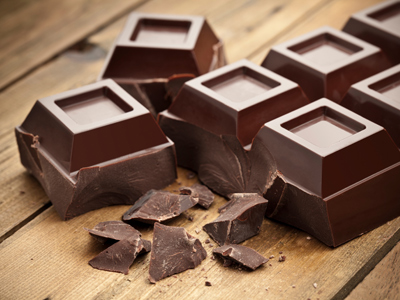What would be a suitable unit to measure the area of a chunk of chocolate?

# Perimeter and Area 2

This Math quiz is called 'Perimeter and Area 2' and it has been written by teachers to help you if you are studying the subject at middle school. Playing educational quizzes is a fabulous way to learn if you are in the 6th, 7th or 8th grade - aged 11 to 14.

It costs only \$12.50 per month to play this quiz and over 3,500 others that help you with your school work. You can subscribe on the page at Join Us

The perimeter of a shape is the distance around its edge. To work out the perimeter of a hexagon multiply the length of one of its sides by 6. If its sides are 2 meters long its perimeter would be 12 meters. The area is the size of the surface. Knowing the area of a wall is important if you are planning to give it a lick of paint - otherwise how will you know how many tins of paint to buy?!

1.
How many cm2 is equivalent to 1m2?
10cm2
100cm2
1,000cm2
10,000cm2
There are 100cm in 1m so 100 x 100 = 1,000
2.
What is the perimeter of a regular pentagon with sides of 5cm?
24cm
100cm
10cm
25cm
A pentagon has 5 sides, therefore 5 x 5cm will give you the perimeter
3.
What is the formula for calculating the perimeter of a rectangle?
2 x length x 2 x width
2 x length + 2 x width
Length + width
Length x width
2 sides of a rectangle are one length whilst the other two sides are a different length, for example 2 sides are 4cm long and the other 2 sides are 6cm long. This rectangle would have a perimeter of 20cm
4.
A regular hexagon has a perimeter of 54cm. What is the length of the sides?
6cm
9cm
12cm
20cm
A regular hexagon has 6 equal sides. To calculate the length of each side, divide the perimeter by 6
5.

Which would be best measured in cm2?

Area of a playground
Area of a town
Area of a desk top
Area of a pen tip
Towns might be measured in km2, playgrounds in m2 and pen tips in mm2
6.
What is the formula for calculating the perimeter of a regular hexagon?
4 x length of side
6 x length of side
6 x length + width
Length of side divided by 6
As a regular hexagon has 6 equal sides, just multiply the length of the sides by 6
7.
Which would be a good estimate of the area of a classroom?
1,000mm2
100mm2
10m2
100m2
10m2 would be large enough for a room in a house but not big enough for a classroom
8.
A rectangle has a perimeter of 38cm. The shortest sides are 7cm. What length are the two longest sides?
12cm
14cm
21cm
28cm
2 x 7 = 14
38 - 14 = 24
24 ÷ 2 = 12
9.
What is the formula for calculating the area of a rectangle?
2 x length x 2 x width
2 x length + 2 x width
Length + width
Length x width
If a rectangle measured 7cm by 10cm its area would be 70cm2
10.
Which would be a suitable unit to measure the area of a chunk of chocolate?
Square grams
Square meters
Square millimeters
Square milliliters
Grams measure weight and milliliters measure volume. Square meters measure area but chocolate bars aren't that big!
Author:  Amanda Swift